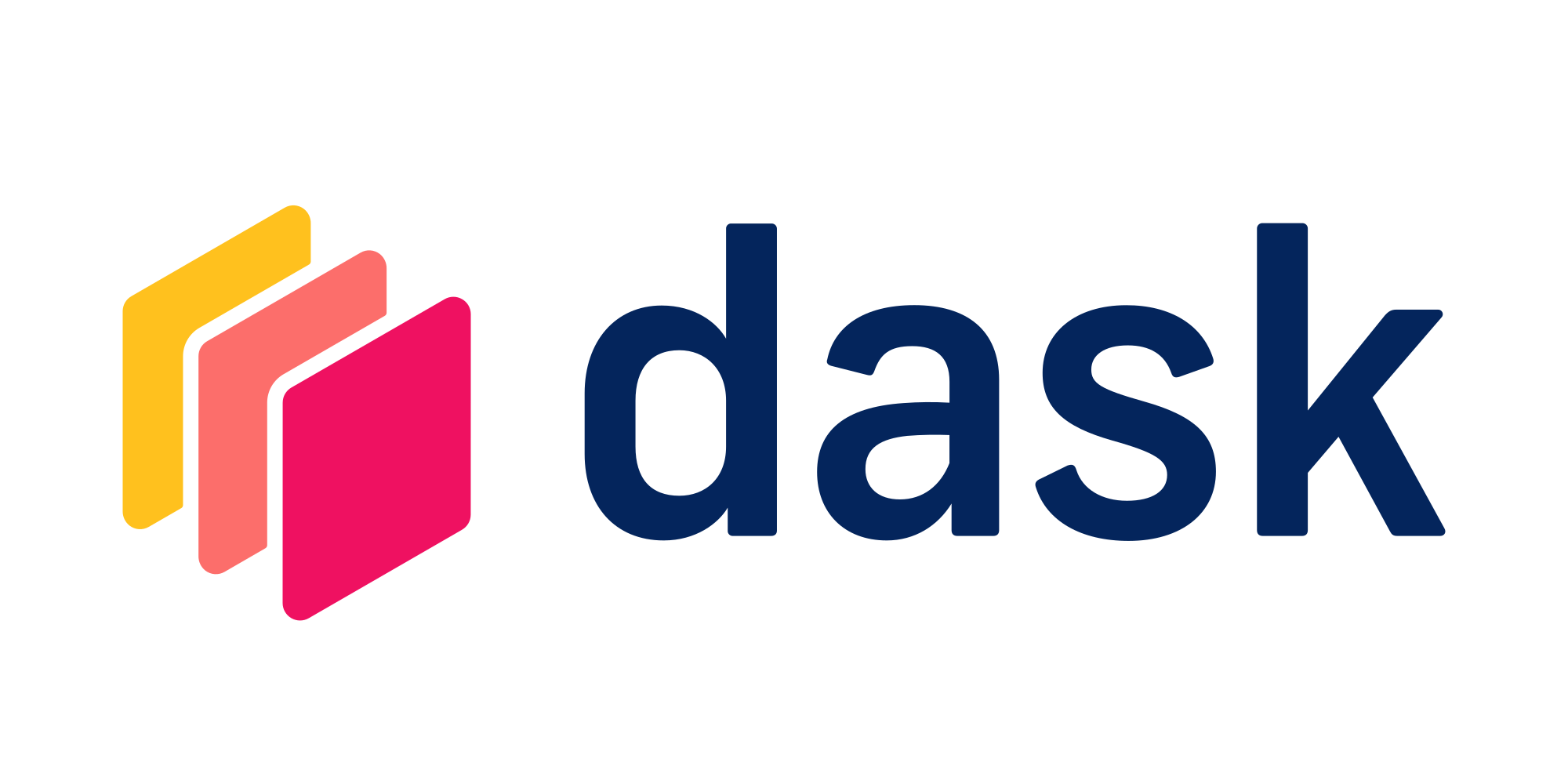Use Dask Bags for distributed sequences of computationsTry this example in seconds on Saturn Cloud

Dask Bags are used for taking a collection of Python objects and processing them in parallel. It can be thought as a sequence of objects that you can do parallel operations on over a distributed cluster. This is similar to the base Python operations like `map`, however the computations will happen in parallel.

A Dask Bag is often a good solution for data that does not fit into a standard tabular or matrix format and thus wouldn’t work with Dask DataFrames or Dask Arrays. For example, a list of nested JSON objects might not be able to easily be converted into a tabular format, but could be converted into a Dask Bag. You can do cleaning and data preprocessing of messy data with a Dask Bag and then at the end convert the data a Dask DataFrame to use in other methods like machine learning model training.

``````from dask_saturn import SaturnCluster

client = Client(SaturnCluster())
``````

After running the above command, it’s recommended that you check on the Saturn Cloud resource page that the Dask cluster as fully online before continuing. Alternatively, you can use the command `client.wait_for_workers(3)` to halt the notebook execution until all three of the workers are ready.

## Create a Dask Bag from a list

In code below we are loading data from a simple Python iteration. Parameter `npartitions` has been set to 2. By default Dask tries to partition data into around a hundred partitions.

``````import dask.bag as db

x = db.from_sequence(list(range(1, 9)), npartitions=2)
``````

Function `read_text` is used to load data directly from a single file or a list of multiple files.

``````x = db.read_text(
[
],
storage_options={"anon": True},
)
``````

## Example using bags

In this example we will load F1 racing data and find the names of F1 drivers whose are ranked 1st through 10th. The data is available from a public URL.

The command below reads the data from the public URL, uses a `map` function to convert each string object in the Dask Bag into a JSON dict. It then takes the first 2 elements of the Dask Bag.

``````import json

b.take(2)
``````

The data is then filtered to the name of driver those who have a rank of at most 10 and the name is pulled.

``````result = b.filter(lambda record: record["rank"] <= 10).map(lambda record: record["name"])
result.compute()
``````

## Best Practices:

Operations involve communication amongst workers (like groups in the data) can be expensive in Dask Bags. Hence if you want to perform operations which involve shuffling of data, it would be advisable to switch to DataFrame or other data type instead.

For more details on using Dask Bags, see the official Dask Bag Documentation.

``````from dask_saturn import SaturnCluster

client = Client(SaturnCluster())

x = db.from_sequence(list(range(1, 9)), npartitions=2)

[
],
storage_options={"anon": True},
)

import json